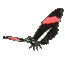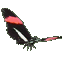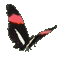Math Help - Divide Fractions - Game Tips:

- The number 8 divided by 2 equals 4 , because (2)(4)=8 in the first place.
- The number 8 divided by the fraction (1/2) equals 16 , because (1/2)(16)=8 .
- The fraction 2/3 divided by the fraction 1/2 equals 4/3 because (1/2)(4/3)=2/3 .

- A standard method to divide two proper fractions, is to multiply the first fraction
by the reciprocal of the second fraction.   [The reciprocal of (c/d) is (d/c)] .

- For example, 1/4 divided by 2/5 = (1/4)(5/2) which equals the answer 5/8 .

- The general rule is   (a/b)/(c/d) = (ad)/(bc) .

- Your Game Score is reduced by the number of butterfly hits.

- To slow the game speed repeat tap/click on the word Slider.
- To increase the game speed repeat tap/click on the word Math.
- Speed can also be adjusted with a keyboard's - and + keys.

- Refresh/Reload the web page to restart the game.